# Vectors Representation

Vectors can be graphically represented by directed line segments. The length is chosen, according to some scale, to represent the magnitude of the vector, and the direction of the directed line segment represents the direction of the vector. For example, if we let 1 cm represent 5 km/h, then a 15-km/h wind from the northwest would be represented by a directed line segment 3 cm long, as shown in the figure at left.

A vector in the plane is a directed line segment. Two vectors are equivalent if they have the same magnitudeand direction.

Consider a vector drawn from point A to point B. Point A is called the initial point of the vector, and point B is called the terminal point. Symbolic notation for this vector is(read “vector AB”). Vectors are also denoted by boldface letters such as u, v, and w. The four vectors in the figure at left have the same length and direction. Thus they represent equivalent vectors; that is,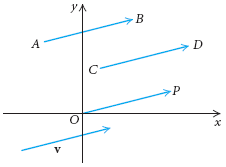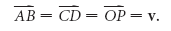In the context of vectors, we use = to mean equivalent.

The length, or magnitude, ofis expressed as ||. In order to determine whether vectors are equivalent, we find their magnitudes and directions.

Example 1 The vectors u,, and w are shown in the figure below. Show that u == w.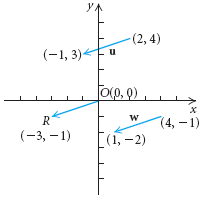Solution We first find the length of each vector using the distance formula:
|u| = √[2 – (-1)]2 + (4 – 3)2 = √9 + 1 = √10,
|| = √[0 – (-3)]2 + [0 – (-1)]2 = √9 + 1 = √10,
|w| = √(4 – 1)2 + [-1 – (-2)]2 = √9 + 1 = √10.
Thus
|u| = |= |w|.
The vectors u,,and w appear to go in the same direction so we check their slopes. If the lines that they are on all have the same slope, the vectors have the same direction.We calculate the slopes: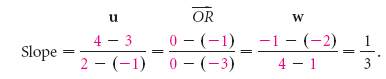Since u,, and w have the same magnitude and the same direction,
u == w.

Keep in mind that the equivalence of vectors requires only the same magnitude and the same direction—not the same location. In the illustrations at left, each of the first three pairs of vectors are not equivalent. The fourth set of vectors is an example of equivalence.

Suppose a person takes 4 steps east and then 3 steps north. He or she will then be 5 steps from the starting point in the direction shown at left. A vector 4 units long and pointing to the right represents 4 steps east and a vector 3 units long and pointing up represents 3 steps north. The sum of the two vectors is the vector 5 steps in magnitude and in the direction shown. The sum is also called the resultant of the two vectors.

In general, two nonzero vectors u and v can be added geometrically by placing the initial point of v at the terminal point of u and then finding the vector that has the same initial point as u and the same terminal point as v, as shown in the following figure.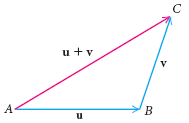The sum is the vector represented by the directed line segment from the initial point A of u to the terminal point C of v. That is, if u =and v =, then
u + v =+=We can also describe vector addition by placing the initial points of the vectors together, completing a parallelogram, and finding the diagonal of the parallelogram. (See the figure on the left below.) This description of addition is sometimes called the parallelogram law of vector addition. Vector addition is commutative. As shown in the figure on the right below, both u + v and v + u are represented by the same directed line segment.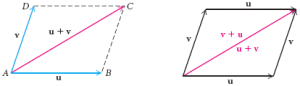If two forces F1 and F2 act on an object, the combined effect is the sum, or resultant, F1 + F2 of the separate forces.### Feedback is important to us.#### Satya Prakash

Mr. Satya Prakash is a research scholar.

error: Content is protected !!